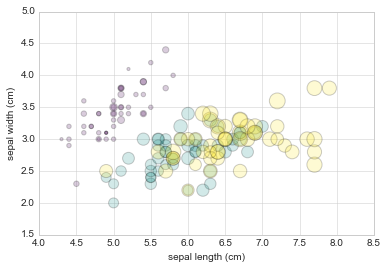# 8.5 简单的散点图

``````%matplotlib inline
import matplotlib.pyplot as plt
plt.style.use('seaborn-whitegrid')
import numpy as np
``````

## 散点图和`plt.plot`

``````x = np.linspace(0, 10, 30)
y = np.sin(x)

plt.plot(x, y, 'o', color='black');
``````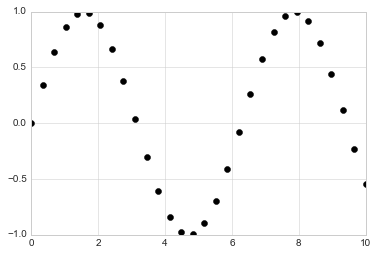``````rng = np.random.RandomState(0)
for marker in ['o', '.', ',', 'x', '+', 'v', '^', '<', '>', 's', 'd']:
plt.plot(rng.rand(5), rng.rand(5), marker,
label="marker='{0}'".format(marker))
plt.legend(numpoints=1)
plt.xlim(0, 1.8);
``````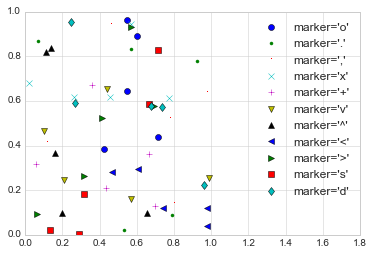``````plt.plot(x, y, '-ok');
``````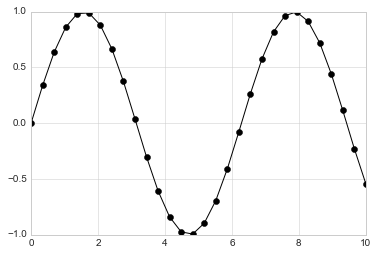`plt.plot`的附加关键字参数，指定了线条和标记的各种属性：

``````plt.plot(x, y, '-p', color='gray',
markersize=15, linewidth=4,
markerfacecolor='white',
markeredgecolor='gray',
markeredgewidth=2)
plt.ylim(-1.2, 1.2);
```````plt.plot`函数的这种灵活性支持各种可能的可视化选项。可用选项的完整说明，请参阅`plt.plot`文档。

## 散点图和`plt.scatter`

``````plt.scatter(x, y, marker='o');
``````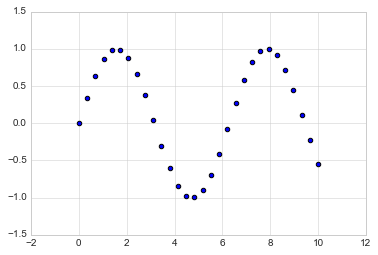`plt.scatter``plt.plot`的主要区别是，它可用于创建散点图，其中每个单独的点的属性（大小，填充颜色，边缘颜色等）可以单独控制，或映射到数据。

``````rng = np.random.RandomState(0)
x = rng.randn(100)
y = rng.randn(100)
colors = rng.rand(100)
sizes = 1000 * rng.rand(100)

plt.scatter(x, y, c=colors, s=sizes, alpha=0.3,
cmap='viridis')
plt.colorbar();  # show color scale
``````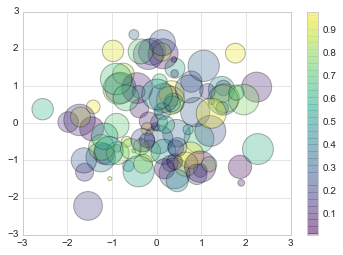``````from sklearn.datasets import load_iris
features = iris.data.T

plt.scatter(features, features, alpha=0.2,
s=100*features, c=iris.target, cmap='viridis')
plt.xlabel(iris.feature_names)
plt.ylabel(iris.feature_names);
``````